Function Repository Resource:

Measure the linear-drift-insensitive, two-sample, phase/frequency stability

Contributed by: Julien Kluge
 ResourceFunction["HadamardDeviation"][data,r,taus] calculates the linear-drift insensitive two-sample deviation of data along sample times taus at rate r.

## Details and Options

The Hadamard deviation is the square root of the linear-drift-insensitive two-sample variance which is used to characterize the frequency/phase stability of an oscillator.
The output usually comes in the format {τ,Around[σ,δσ]} where δσ denotes the statistical uncertainty related to the deviation σ.
The argument taus can be specified as:
 {{n}} attempts to place n logarithmic spaced samples d places logarithmic spaced samplings with log base d {τ1,τ2,…} tries to use the specified sample times All uses all possible sample times Automatic tries to place a reasonable amount of samples along the whole possible time range
For a list of reals as a data argument, ResourceFunction["HadamardDeviation"] will use a fast, compiled algorithm. Otherwise valid inputs are exact expressions and/or Around.
The rate r must be a positive, numeric quantity.
Supported options are:
 "Overlapping" True whether to use the overlapping Hadamard deviation "FrequencyData" False specifies whether the data argument is a list of frequencies instead of phase data

## Examples

### Basic Examples (3)

Calculating the Hadamard deviation for a small sample set at a rate of 2 samples per time unit:

 In:=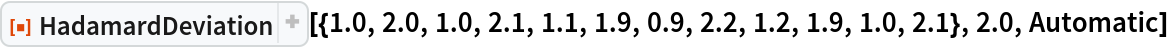Out=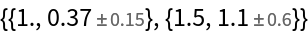Display overlapping Hadamard deviation of white noise at a rate of 0.1 for automatically chosen sampling points:

 In:=Out=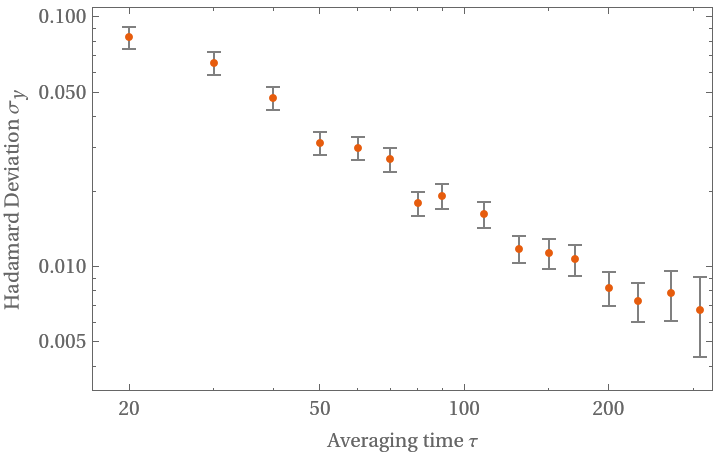Display overlapping Hadamard deviation of white noise at a rate of 0.1 for certain sampling points:

 In:=Out=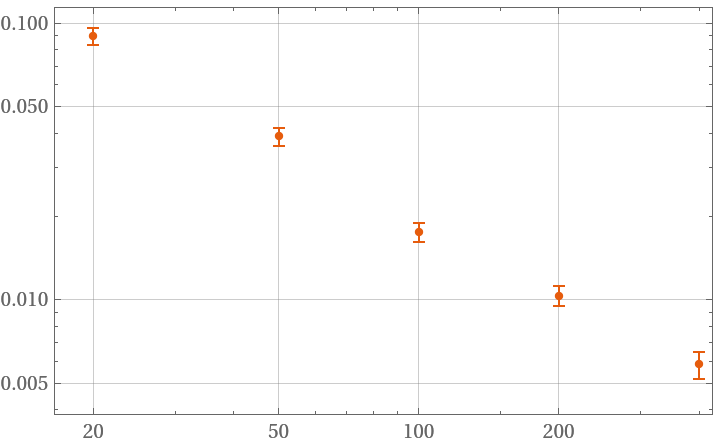### Scope (3)

Sample at all possible times:

 In:=Out=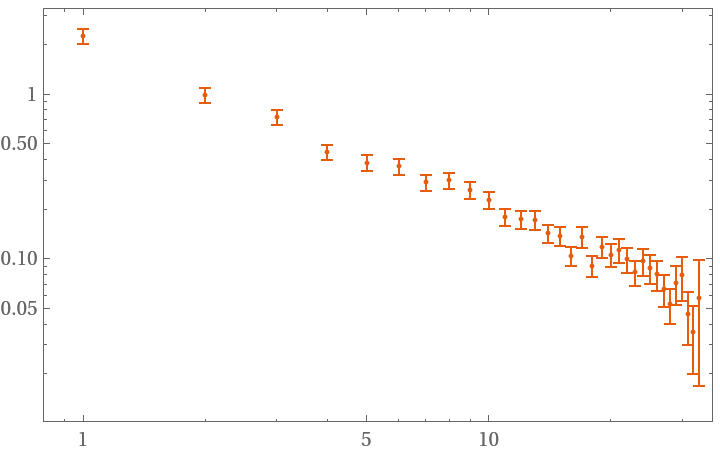Try to sample at 4 different time values:

 In:=Out=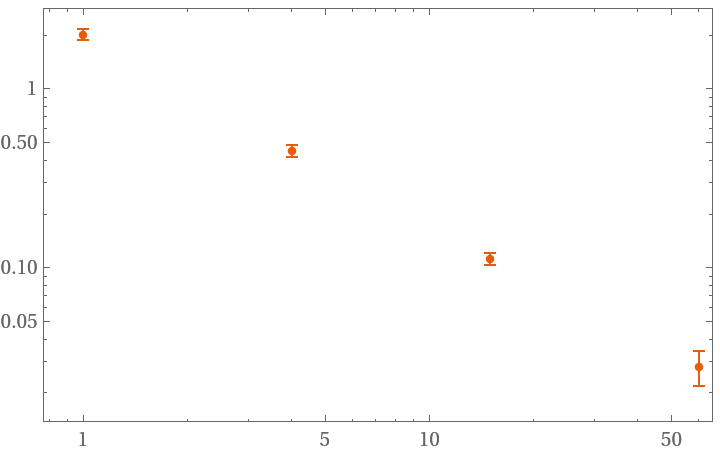The function will try to use a fast evaluation method when the data array is fully real and otherwise prints a warning:

 In:=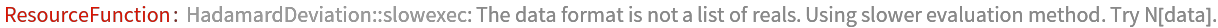Out=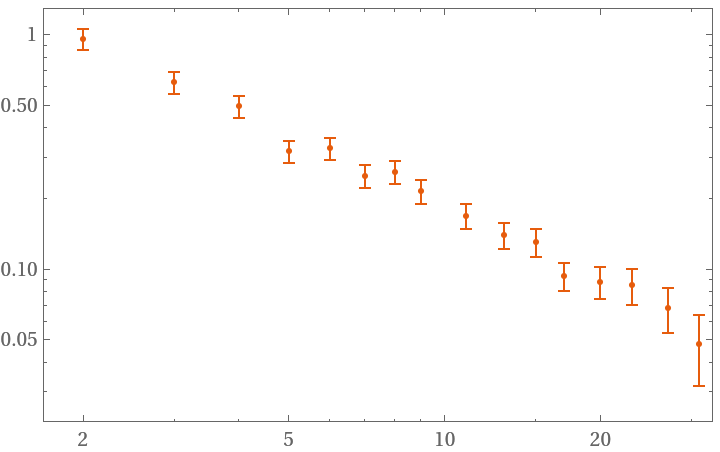### Options (2)

#### FrequencyData (1)

FrequencyData accepts a Boolean as argument. True signals the use of frequency data as opposed to phase data:

 In:=Out=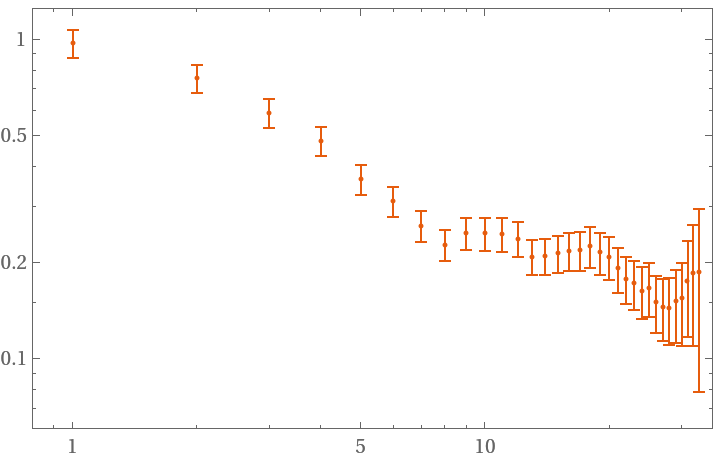#### Overlapping (1)

Overlapping accepts a Boolean as argument. False signals the use of non overlapping strides in the deviation sampling:

 In:=Out=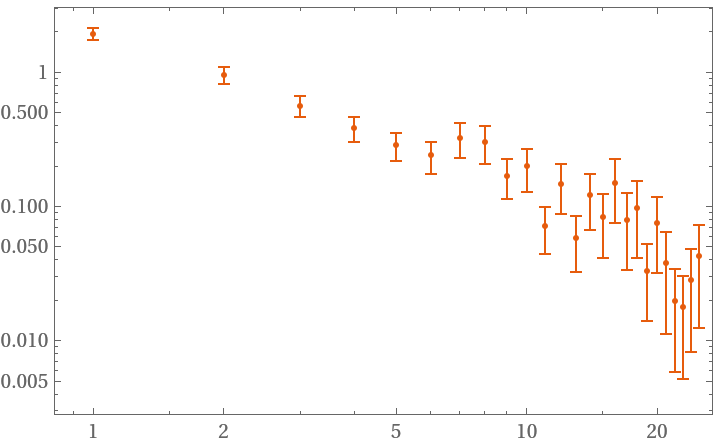### Applications (2)

Distinguish random walk from white noise by their distinct slope:

 In:=Out=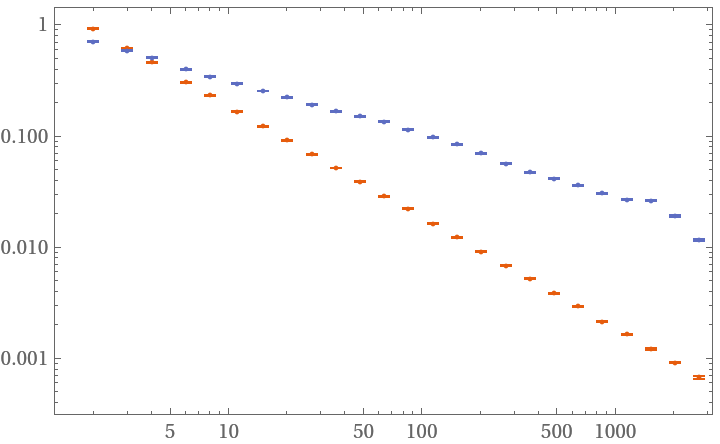The Hadamard deviation is is approximately equal to the Allan deviation except for linear drifts:

 In:=Out=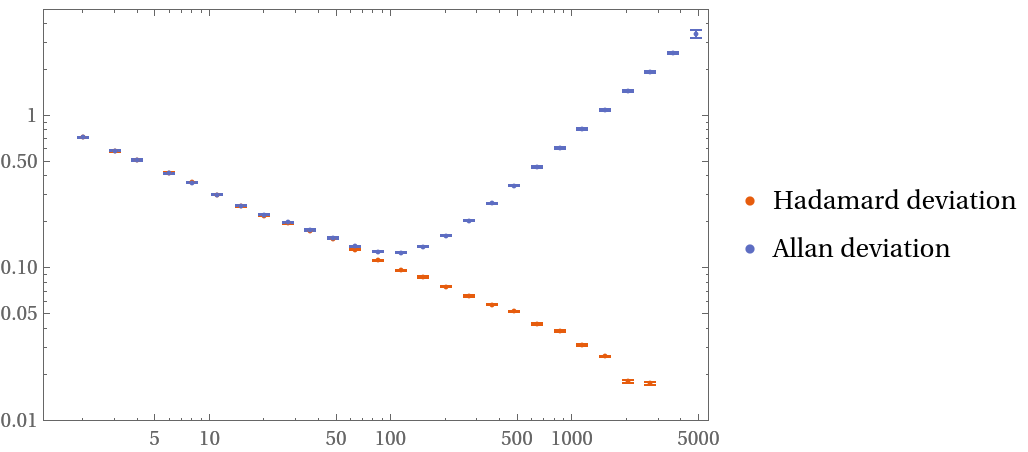### Properties and Relations (1)

Using the fast algorithm is approximately 2 times faster:

 In:=Out=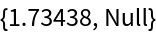In:=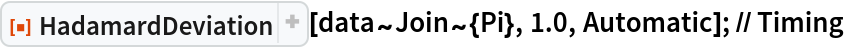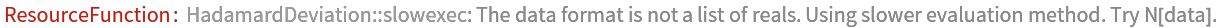Out=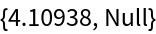### Possible Issues (1)

Requesting a certain amount of samples will not necessarily result in this amount of deviations:

 In:=Out=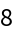Julien Kluge

## Version History

• 1.0.0 – 01 February 2021